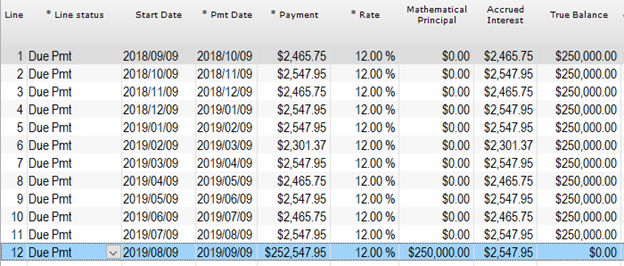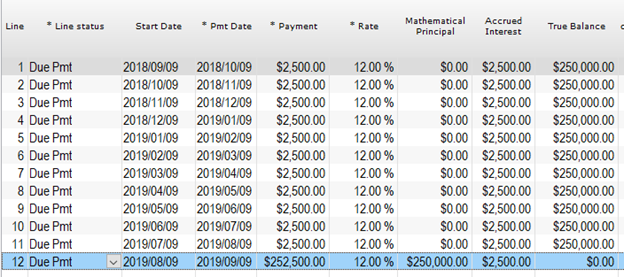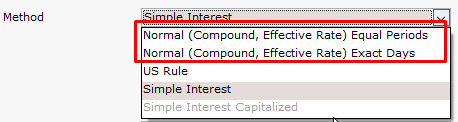# Interest-only: Regular monthly interest vs. Exact day interest

Question:

My company does interest-only 12 month bridge loans calculated in two ways.

1. Payments are based on the number of days in a month with a balloon payment at the end (so payments change depending on the month)
2. Each of the 12 payments is equal with a balloon payment at the end.

Can these two calculation methods be done in Margill?

Yes.

In Simple interest, Margill will usually use the exact number of days in a month and in a year to compute the interest. The Day count would be Actual/Actual (or Actual/365 or Actual/360).If the interest is to be the same every month, the use the 30/360 Day count which simulates months that are of the same length.For Compound interest (what is called the Effective rate method – the banking method), there is an extra  calculation method option that calculates using the exact number of days and another that splits payment in equal periods. The Day count does not have to be used to “artificially” simulate the equal periods.